# Lecture 1: The Real Numbers

## 1.1 What Are They?

This course will deal with multivariate calculus, basically the analytical study of regions of n-dimensional real space. Before considering this, we should review what we mean by the term 'real numbers'.

The basic idea is that the real numbers are the elements of the set of real numbers. However, a set is just a set; something about which we can take subsets and do other set theoretic operations. In order to understand this, we need to consider the set of real numbers as something with more structure. The set of real numbers is actually a complete ordered field.

Let us pause to describe what this means:

Definition 1: A field is a triple (F, +, *) where F is a set and + and * are binary operators (called addition and multiplication) which satisfy the following axioms:

1. Both addition and multiplication are commutative and associative and multiplication is distributive over addition. In symbols, this means that for all a, b, and c in F, one has:
1. a + b = b + a, a*b = b*a
2. (a + b) + c = a + (b + c), (a*b)*c = a*(b*c)
3. a*(b + c) = a*b + a*c
2. (Identities) There are two different elements 0 and 1 (called zero and one) in F such that for all a in F: a + 0 = a and a*1 = a.
3. (Inverses) For every a in F, there is at least one b in F such that a + b = 0. Further, if a is non-zero, there is at least one c in F such that a*b = 1. The elements b (respectively c) are called the additive (respectively the multiplicative) inverses of a and are denoted -a (respectively 1/a).

Definition 2: An ordered field is a pair ((F, +, *), <) where (F, +, *) is a field and < is binary relation on F such that:

1. (Transitivity) For every a, b, c in F, if a < b and b < c, then a < c.
2. (Trichotomy) For every a and b in F, exactly one of the following holds:
1. a < b
2. a = b
3. b < a
3. For all a, b, and c in F, if a < b, then a + c < b + c. Further, if c > 0, then a*c < b*c.

It is assumed that you have probably already had a course in which you showed that most of the standard rules of algebra follow from the assumption that one has an ordered field. (If you haven't ever done this before, then take home and work through Landau's Foundations of Analysis; he will show you in a weekend read how to derive the basic properties of the real numbers starting from Peano postulates. Just for practice, however, you might try to show:

Exercise 1 In any field, additive and multiplicative identities and inverses are unique. In an ordered field, if a < b and c < 0, then b*c < a*c.

Definition 3: A subset S of an ordered field F is bounded above by an element a in F if b < a for all b in S. The upper bound a is called a least upper bound for S if every other upper bound of S is larger than a.

Definition 4: An ordered field F is said to be complete if every subset S of F which has an upper bound, also has a least upper bound.

Exercise 2: Show that least upper bounds are unique. Mimic the above definition to define lower bounds and greatest lower bounds. Show that in complete fields, every set S with a lower bound has a greatest lower bound.

It is useful to have the notion of complete ordered field in order to sort out some basic properties of real numbers. But the real value is that these assumptions alone are enough to completely characterize the real numbers. The result is expressed in terms of:

Definition 5: An order isomorphism between two ordered fields ((F,+,*),<) and ((G,+,*),<) is a 1-1 and onto map f:F ->G such that for all a and b in f, one has:

1. f(a + b) = f(a) + f(b)
2. f(a*b) = f(a)*f(b)
3. If a < b, then f(a) < f(b).

So basically, two ordered fields are order isomorphic if we cannot distinguish between them by using their addition and multiplication operations and their order relation. In these terms the basic result is:

Theorem 1: There is a complete ordered field and any two complete ordered fields are order isomorphic.

This result will not be proved here, but is found in algebra books. Here are some of the ideas of a proof:

1. Using the elements 0 and 1, one can build up the integers through addition, and then one can take quotients to get the rational numbers Q.
2. Define a sequence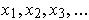of elements of an ordered field to be a Cauchy sequence if for every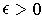, there is an n such that for any i, j greater than n, one has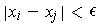. We say that the sequencehas limit x if for every, there is an n such that for every i > n, one has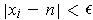.
3. Two sequencesand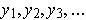are said to be equivalent if the sequence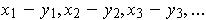has limit 0. You can show that this is an equivalence relation.
4. Ifandare Cauchy sequences, then adding or multiplying corresponding terms gives new Cauchy sequences. Similarly, taking additive inverses gives a Cauchy sequence and so does taking multiplicative inverses if the original sequence has a non-zero limit and none of the elements are zero.
5. So that equivalence is preserved under addition and multiplication of Cauchy sequences.
6. Take the set S of Cauchy sequences with elements in the rational numbers Q. The set of equivalence classes of such sequences can be shown to be a complete ordered field.

## 1.2 The Cauchy-Schwartz Inequality

Henceforth, let use let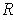denote the real numbers, i.e. the set part of a complete ordered field. By taking n-tuples of real numbers we get n-dimensional real space, denoted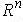; its elements are called points. The points are demoted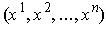or simply (x^i). Points can be added componentwise and we can multiply a real number by a point to get another point whose coordinates are just the original coordinates multiplied by the real number. Of course, this makesinto an n-dimensional vector space. But, we can also define a dot product: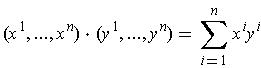As usual, this allows us to define the length of a point as the square root of the dot product of the point with itself: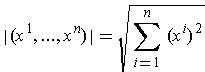Now the length function behaves like you would expect. For example:

Proposition 1 (Triangle Inequality) For any two points x and y in, one has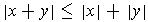Proof: Squaring both sides, we see this amounts to showing that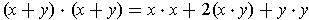is at most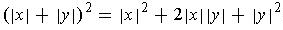Comparing terms, we see that the triangle inequality is equivalent to:

Proposition 2 (Cauchy-Schwartz Inequality) For any two points x and y in, one has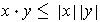, i.e.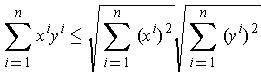Exercise 3: Draw a picture in the case n = 2 and identify various factors of the left hand side divided by the right to convince yourself that the result is basically equivalent to the addition formula for cosines.

Recall from your calculus course, that the dot product was shown to be: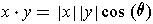where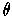is the angle between the vectors from the origin to the points x and y respectively. This was probably only shown in the case n = 2; but it is another way of understanding why Proposition 2 should be true. In our case, we will actually do things in the opposite direction, i.e. prove that Proposition 2 is true and use it to define the angle.

The main use of the dot product in Calculus was to give you the means of calculating the projection of one vector on another. Using this interpretation, can you see why Proposition 2 should be true?

Given two vectors (i.e. two points) x and y, if we subtract from x the vector projection p of x on y, then we get a vector perpendicular to y. Now, geometrically, this vector should be smallest vector amongst all vectors of the form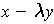for all possible real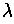. Geometrically, we have `solved' the problem: Minimize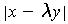.

Ahh! we are finally doing calculus!

So let's do calculus: To minimize this, we should minimize the square,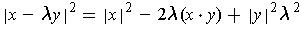If x or y is zero, this is easy (What is the answer?); so assume both are non-zero. Now, if think of x and y as being constants, then this is just a parabola. So, either from your knowledge of parabolas or from elementary calculus, it is easy to see when this is minimized: Setting the derivative to zero gives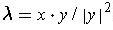. Substituting back shows that the minimum value is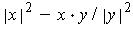. Since this minimum value is non-negative, we have just proved Proposition 2.

A slight variant: We know that the expression on the right side of the displayed formula must be positive unless x is a multiple of y. But, if it is positive for all values of, then the roots must both be complex and so the discriminant is negative. But this is precisely the Cauchy-Schwartz inequality.

Remark: Make sure you sort out all the geometry from the proof to be sure we really have a proof here and not just heuristic hand waving. When you have done this, compare to the proof in Spivak -- hopefully, then you will understand his very slick proof.

Another approach entirely Let's go back to the notion of angle between two vectors. Along with the dot product, you had a vector cross product in the case of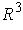. It gave you a vector that was perpendicular to the original two vectors, say v and w, and its length was precisely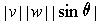. It could also be calculated as the value of the determinant of a strange looking matrix in which the top row had the unit vectors i, j, and k in the coordinate axis directions and the other two rows had the coordinates of v and w respectively. Remember the formula: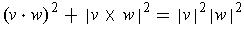(If not, then it is time to prove it -- yes, the Pythagorean Theorem in its many guises, including trigonometry, is your best friend.)

Identities are mysterious and wonderful. If you write the above in terms of all the coordinates, then you simply have an algebraic identity.

Exercise 4: Use the above identity to prove the Cauchy-Schwartz inequality. Now generalize it to n dimensions and get another proof of Proposition 2.